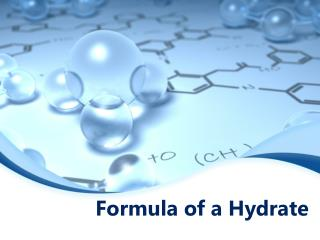DownloadDownload PresentationFormula of a Hydrate

# Formula of a Hydrate

Télécharger la présentation## Formula of a Hydrate

- - - - - - - - - - - - - - - - - - - - - - - - - - - E N D - - - - - - - - - - - - - - - - - - - - - - - - - - -
##### Presentation Transcript

1. Formula of a Hydrate

2. What is a hydrate? An ionic compound that contains water molecules in its structure The formula states how many mol of water molecules per one molof ionic compound Example: CuSo4 5 H20 Copper sulfate pentahydrate

3. What is an anhydrate? The substance that remains after the water is removed from a hydrate Example: CuSo4 Anhydrous Copper sulfate

4. Prefixes

5. Steps to find formula • Identify what’s given: • Hydrate/anhydrate (go to step 2) • Anhydrate/water (skip to step 3) • If given hydrate and anhydrate, subtract to get water • Convert mass of anhydrate and water to moles of each, respectively • Find the ratio of water to anhydrate (in moles) • Write the formula; ionic compound followed by ratio of water • Name the compound

6. Example 1 A calcium chloride hydrate has a mass of 4.72g. After heater for several minutes the mass of the anhydrate is found to be 3.56g. Determine the formula and name of the hydrate. Calcium chloride: Ca2+Cl− CaCl2 Step 2: 4.72g hydrate − 3.56g anhydrate 1.16g H2O Step 3: 3.56g CaCl2 1mol CaCl2 110.98g CaCl2 1.16g H2O 1mol H2O 18.02g H2O = 0.0321 molCaCl2 = 0.0644 molH2O SIG FIGS IN ANSWER MUST MATCH GIVEN!!

7. • 1 Step 4: Ratio 0.0644 mol H2O2 0.0321 mol CaCl2 Step 5: CaCl22H2O Step 6: calcium chloride dihydrate

8. Example 2 What is the formula an name for a hydrate which consists of 76.9% CaSO3 and 23.1% H2O? Step 3: 76.9g CaSO31mol CaSO3 110.98g CaSO3 23.1g H2O 1mol H2O 18.02g H2O = 0.640 mol CaSO3 = 1.28 mol H2O SIG FIGS IN ANSWER MUST MATCH GIVEN!!

9. Step 4: Ratio 1.28 mol H2O2 0.640 mol CaSO3 Step 5: CaSO32H2O Step 6: calcium sulfite dihydrate ≈ • 1